Courses

# Chapter 3 - DC Machines (Part - 3) - Notes, Electrical Machines, Electrical Engineering Electrical Engineering (EE) Notes | EduRev

## Electrical Engineering (EE) : Chapter 3 - DC Machines (Part - 3) - Notes, Electrical Machines, Electrical Engineering Electrical Engineering (EE) Notes | EduRev

The document Chapter 3 - DC Machines (Part - 3) - Notes, Electrical Machines, Electrical Engineering Electrical Engineering (EE) Notes | EduRev is a part of the Electrical Engineering (EE) Course Electrical Machines for Electrical Engg..
All you need of Electrical Engineering (EE) at this link: Electrical Engineering (EE)

MAGNETISATION CURVE

The magnetisation curve is the relationship between air-gap flux Ø and the field winding Mmf or field winding current.

• For constant speed ωm, the armature generated emf Ea at no-load, is proportional to air-gap flux Ø only.
• This curve is also called no-load or open circuit characteristic, or saturation curve.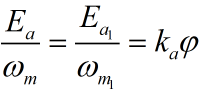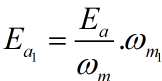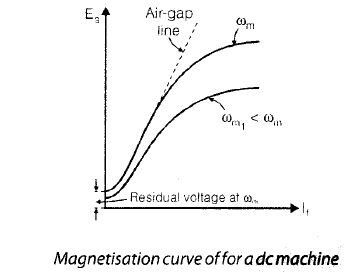• The magnetisation cure for any other speed, can be obtained by equation.
• The dashed straight line, tangent to the straight line portion of magnetization curve at speed ωm , is called air-gap line.

OPERATING CHARACTERISTICS
DC Generators

• More important characteristics of dc generators are four and these are given below :

Ea = f (If) with speed n = constant. This characteristic is also called saturation curve, open-circuit characteristic, magnetization curve or no-load magnetization curve.

Vt = f (If) with both Ia and n constant. This characteristic is also called magnetization curve.
External characteristic
Vt = f (IL) with both If and n constant.

Armature characteristic or regulation curve

If = f (Ia) with both Vt and n constant

•  The shape of these characteristics depends upon the method of exciting the field winding.

Remember :

• All the dc generator characteristics listed above, speed is held constant by the prim-mover
• These are generally four types which are given below. These are different for different types of d.c. generator.

Separately-Excited Generator

These generators are used when a wide range of output voltage is required.

• This characteristic gives the variation of armature generated e.m.f. Ea with field current If, for zero armature current and constant speed.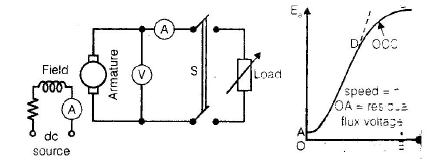• The magnetization curve for low values of If is a straight line, because the entire field mmf is almost spent in forcing the flux through the air gap and the mmf required by the iron is almost negligible.
• With increased values of If and above a certain value of field flux, saturation sets in and mmf required by the iron increases more rapidly than the flux.

• Run the armature at constant rated speed and close the switch S.
• Adjust the field current till Ia is equal to rated armature current (or any other specified current) and take the instrument readings.
• Vary the load an field currents in such a manner that armature current Ia and speed n remain constant, but terminal voltage Vchanges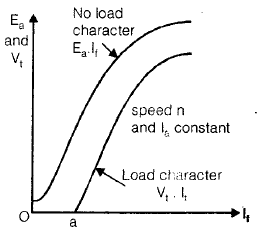External characteristics

• The generator is run at rated speed and its field winding is excited to give rated terminal voltage at no load. Now close the switch S, vary load resistance in steps and for each step, note terminal voltage and load current. A typical external characteristic is shown in figure (b), by curve 1.
• The decrease in terminal voltage drops caused by armature reaction and armature circuit resistance.
• The internal characteristic gives the variation of armature no-load generated emf Ea minus the minus the voltage drop due to armature reaction, with load current IL or Ia.
• Internal characteristic gives the relationship between internally generated emf and the load current for constant speed and field current.
• OA is the no-load terminal voltage Ea.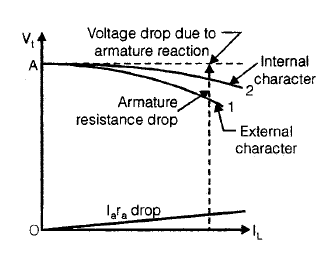Armature Characteristic or Voltage Regulation

• At no load, the field current is adjusted to give rated terminal voltage of the machine, figure (a). The load current is now increased in steps and at each step, the field current is adjusted to keep the terminal voltage constant. The regulation curve is then plotted as shown in figure. In the beginning, the curve is almost a straight line and as the saturation sets in, it bends upwards.
• This characteristic can be employed for calculating the number of series turns for any degree of compound in dc compound machines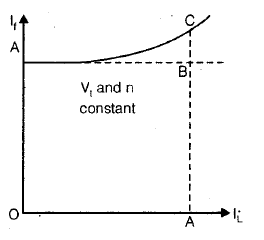Shunt Generators
Remember :

• The load current must be well below the maximum current for avoiding large dips in the terminal voltage.

• If the field winding circuit of the shunt generator is disconnected from the armature circuit and separately excited, then the no-load characteristic with separate excitation will not difference from that obtained with shunt excitation.
• This is due to the fact that small amount of current (1 to 3% of rated current) flowing in the armature of the shunt generator, has negligible effect on the main flux.

• The load characteristics obtained both for separate and shunt excitation, are the same.
• The slight difference is due to the different armature currents, Ia = IL + If for shunt generator and Ia = IL for separately - excited generator.

External Characteristic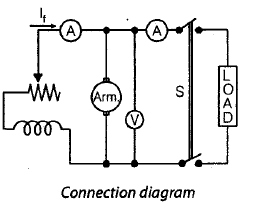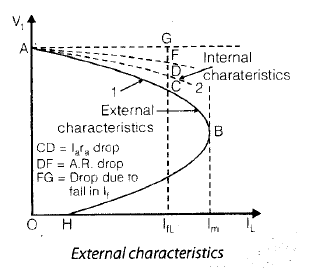• The generator is run at rated speed and the field current is adjusted to give rated volt age (or any other suitable voltage) at no-load. Switch S is closed, the load is gradually increased in steps and the instrument readings at each step are recorded. A plot of the terminal voltage Vand load current IL, with present value of field current and speed, gives the external characteristic curve 1 in figure.
• The drop in terminal voltage is due to (a) armature resistance drop Ia ra (b) reduction in main flux due to armature reaction and (c) further reduction in field current It.
• A reduction in field current causes the flux and therefore the generated emf to decrease. Thus the terminal voltage of the generator for a given load current, will be lower when shunt excited than when current, will be lower when shunt excited than when separately excited, provided no load voltage is same in both the cases.
• Over the normal operating range, the internal characteristic given by curve 2, can be obtained by adding Ia rdrop to the ordinates of external characteristic.

Armature Characteristic

• Shunt generator armature characteristic is obtained by running it as a separately excited generator.

Voltage Building in Shunt Generator

• Consider an unloaded shunt generator as illustrated in figure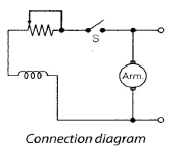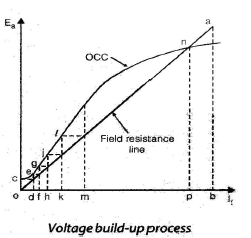• The straight line Oa is the graphical plot of Ohm’s for the field circuit. In other words, the slope of the line Oa, drawn through origin, represents the field resistance.
• When the armature is driven at a speed flow which the magnetization curve is given, the residual pole flux generates a small voltage Oc, with switch S open, i.e. with zero field current. when switch S is closed, residual flux voltage Oc produces a small field current. If the flux produced by this small field current, adds to the residual flux, still larger voltages are generated.
• Residual flux voltage Oc is shown to produce a small field current equal to Od, which in turn raises the generated voltage to de. This voltage de, raises the field current to Of, which further raises the generated emf to fg, now emf fg raises the field current to Oh, which in turn increases the generated emf to hj and so on, till stable point n is reached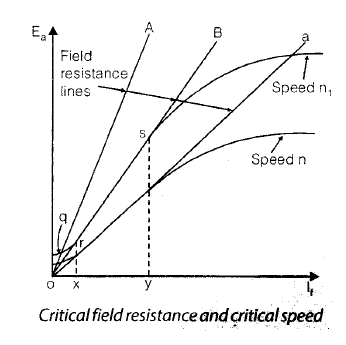• If shunt field resistance is increased to OA (slope of the field resistance line increased) then the field resistance line and the magnetization curve intersect at point q, therefore, the voltage will not build up further than point q.
• Field resistance line OB is tangent to the magnetization curve and the field resistance represented by line OB is called the critical field resistance at a given speed.
• If shunt field resistance is more than the critical field resistance such as given by line OA, there will be no voltage build up.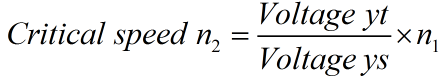• Critical speed is the speed at which the dc shunt generator just fails to build no external resistance in field circuit.

Failure to Build-up

• If the self-excited generators fail to build-up the voltage at no-load, it may be due to any one of the following reasons.

No-residual Magnetism

• The voltage build up process can start only if there is residual magnetism in the magnetic circuit of the generator.

Field Connections Reversed

• If the field-connections are incorrect, the flux produced by small field current opposes the residual flux and the generated voltage decreases to zero.

High Field Circuit Resistance

• In case the field resistance is more than the critical field resistance, voltage will not build up. Speed Less than Critical Speed
• The armature speed being less than the critical speed.

Series Generator

These generators are used mainly as series boosters connected in the line, to neutralise the effect of line ohmic drop.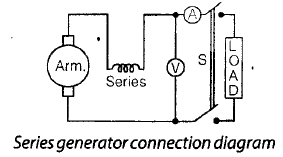• In a series generator, the armature winding, field winding and load resistance are connected in series, therefore the field current is equal to the armature or load current.
• The no-load magnetization curve can only be obtained by separately exciting its field from a low voltage source.
• The magnetization curve at one speed for a series generator is illustrated by curve 1 in figure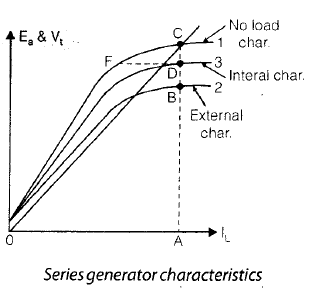• This characteristic also, can only be obtained by separately exciting the series field winding. The shape of this characteristic is identical with that of the separately excited generator.

External Characteristic

• With the switch S open, the small voltage due to residual flux will be indicated by the voltmeter. When the switch S is closed, field current equal to the load current, starts flowing. If the current in the series field produces a flux aiding the residual flux, the generator will build up voltage till point C is reached.
• The field resistance line OC depends on the total resistance in the series circuit i.e., the slope of line OC is determined by the sum of armature circuit resistance, series field resistance and load resistance.
Offer running on EduRev: Apply code STAYHOME200 to get INR 200 off on our premium plan EduRev Infinity!

## Electrical Machines for Electrical Engg.

22 videos|41 docs|26 tests

,

,

,

,

,

,

,

,

,

,

,

,

,

,

,

,

,

,

,

,

,

,

,

,

,

,

,

;